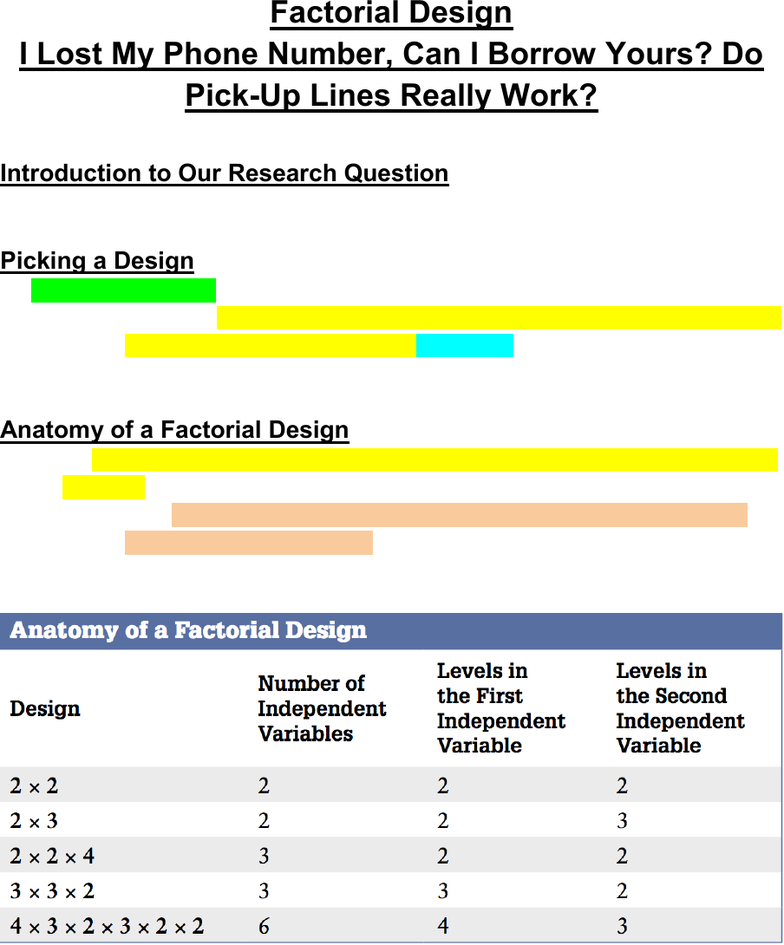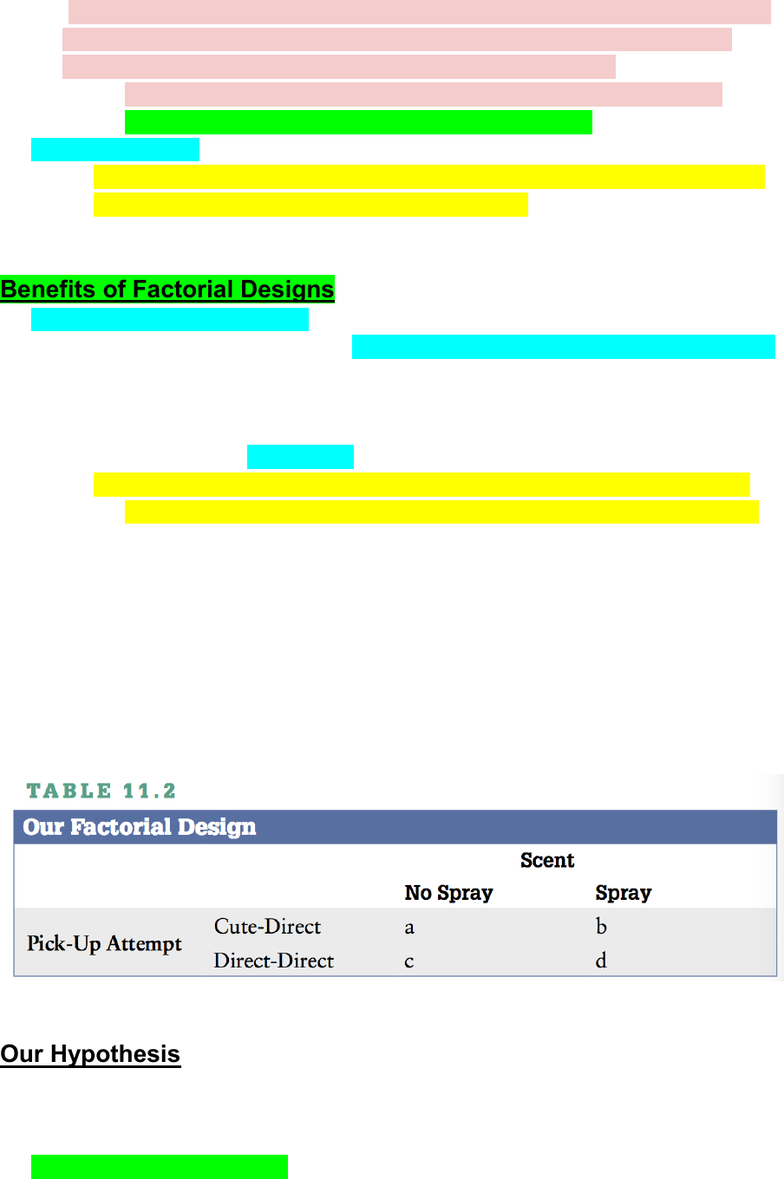Textbook Notes (290,000)
CA (170,000)
UTSC (20,000)
Psychology (10,000)
PSYB01H3 (600)
Anna Nagy (200)
Chapter 11

# PSYB01H3 Chapter Notes - Chapter 11: Dependent And Independent Variables, Statistical Hypothesis Testing, Energy Drink

Department
Psychology
Course Code
PSYB01H3
Professor
Anna Nagy
Chapter
11

This preview shows pages 1-2. to view the full 8 pages of the document.Factorial Design
I Lost My Phone Number, Can I Borrow Yours? Do
Pick-Up Lines Really Work?
Introduction to Our Research Question
“ How do pick-up lines and a person’s scent influence relationship initiation?”
Picking a Design
factorial design
the most frequently used experimental design for a study that has more
than one independent variable. + LEVELS
in this case → pick up line and scent
Anatomy of a Factorial Design
the number of numbers indicates how many independent variables the design
includes.
e.g. In a 3 × 4 factorial design, there are two numbers, so there are
two independent variables.
e.g. In a 2 × 2 × 4 factorial design, there are three numbers, so there
are three independent variables.
numbers themselves indicate how many levels or conditions each
independent variable has.
e.g. In a 3 × 4 factorial design, the first independent variable has 3
levels, while the second independent variable has 4 levels.
a 5 × 3 × 2 × 4 × 2 factorial design is theoretically possible, it is not

Only pages 1-2 are available for preview. Some parts have been intentionally blurred.factorial designs are true experiments, where the researcher manipulates the
independent variables, all of which are between-subjects, such that each
participant only gets one level of each independent variable.
however, if one of the independent variables use a within-subject
format → factorial design becomes a mixed design
hybrid design
any factorial design that has at least one quasi- independent variable.
a variable you cannot manipulate → gender
Benefits of Factorial Designs
establish cause and effect!
allowing the researcher to run multiple experiments for the price of one
e.g. a 2 × 2 factorial design = two-group design
e.g. If we had a 2 × 3 factorial design, it would be like combining
a two-group design with a multi-group design
it allows us to know interaction
when one independent variable influence on the dependent variable
changes depending on the level of the other independent variable(s).
e.g. If you were to consume too much of an energy drink, you would
become hyper alert, edgy, and jittery. But combined with alcohol, the
effect of the energy drink on these outcomes is different.
One independent variable (Red Bull) alters how the other
independent variable (alcohol) influences the dependent
Our Hypothesis
“How do pick-up lines and a person’s scent influence relationship initiation?
to answer this question we need multiple independent variables =
multiple hypothesis
main effect hypothesis

Unlock to view full version

Only pages 1-2 are available for preview. Some parts have been intentionally blurred.focuses on one independent variable at a time, ignoring all other
independent variables.
e.g. In our study we have two potential main effect hypotheses, one
for pick-up approach and one for scent
multiple independent variables can also mean we have interaction effect
hypothesis
prediction about how the levels of one independent variable will
combine with another independent variable to impact our dependent
variable in a way that extends beyond the sum of the two separate
main effects.
e.g. In our study we believe pick-up attempt and scent combined can
influence relationship receptivity, so we have a potential interaction
between our two independent variables.
In factorial designs, you have as many potential main effect hypotheses as
you have independent variables, and as many interaction hypotheses as you
have combinations of independent variables.
main effect and interaction hypotheses are not dependent on one
another.
That is, you can have an interaction hypothesis without predicting
differences for either main effect.
Or you could have a main effect hypothesis for one independent
variable without having a main effect hypothesis for the other
independent variable.